• Call Now

1800-102-2727•

# Law of Conservation of Momentum Derivation

The momentum of an item is the product of its mass and velocity. It is a scalar quantity. The conservation of momentum is a fundamental rule. It asserts that the entire momentum of an isolated system is conserved. In other words, if no external force occurs on the system, the overall momentum of a system of objects remains constant during any interaction. The vector sum of individual momenta is the overall momentum. As a result, the component of total momentum in any direction remains constant. In any physical process, momentum is preserved.

## Illustration in a Single Dimension

A one-dimensional collision of two objects can be used to demonstrate momentum conservation. Two objects with masses m1 and m2 collide while travelling in a straight line at velocities u1 and u2, respectively. They acquire velocities v1 and v2 in the same direction as a result of the collision.

Before collision, total momentum can be written as: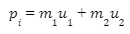After collision, total momentum can be written as: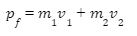Total momentum is preserved if no other force operates on the system of the two objects. Therefore,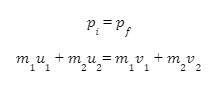## Derivation of Momentum Conservation

If no external force is applied to the system of two colliding objects, the objects apply impulse to each other for a brief moment at the point of contact. The impulsive force exerted by the first object on the second object is equal and opposite to the impulsive force applied by the second object on the first object, according to Newton's third law of motion.

During a one-dimensional collision between two objects with masses m1 and m2, with velocities u1 and u2 before the collision and velocities v1 and v2 after the collision, the impulsive force on the first object is F21, and the impulsive force on the second object is F12. Using Newton's third rule, we can see that these two impulsive forces are equal and opposing, i.e.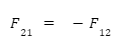The impulse of the force F21 is equal to the change in momentum of the first item if the time of contact is t.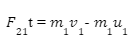The change in momentum of the second item is equal to the impulse of force F12.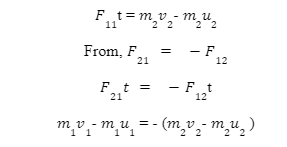According to this relationship, momentum is preserved throughout the collision.

## Examples of Momentum Conservation

A gun's recoil: When a bullet is shot from a gun, both the bullet and the gun are initially at rest, resulting in a total momentum of zero prior to firing. When the bullet is discharged, it gains forward motion. The cannon gains reverse motion as a result of momentum conservation. A bullet with mass (m) is fired at a forward velocity of ‘v’. The mass (M) of the gun obtains a rearward velocity ‘u’. Because the total momentum is zero before firing, the total momentum after firing is also zero.
0 = mv + Mu
u = m/Mv

The recoil velocity of the gun is denoted by ‘u’. The mass of the bullet (m) is much less than that of the gun (M).
i.e. M >> m.
The backward velocity of the gun is minute,
v >> u

Rocket Propulsion: At one end of a rocket, there is a gas chamber from which gas is discharged at high velocity. The overall momentum is zero prior to the ejection. The rocket gains recoil velocity and acceleration in the opposite direction as a result of the gas ejection. This is the result of momentum conservation.

If a rocket of mass ‘m’ ejects a propellant of minor mass dm with an exhaust velocity ve such that the remaining rocket of mass m - dm obtains a velocity ‘dv’ in the opposite direction, the propellant and residual rocket momenta are equal in magnitude and opposite in direction.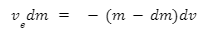Because ‘dm’ and and ‘dv’ are both tiny, the equation may be approximated as: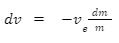If there is reduction in the mass of the rocket from m0 to m as the velocity of the rocket increases from 0 to v, then upon integration of above equation: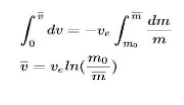## What Did You Know?

• Photons, which are massless particles, make light. A photon, on the other hand, possesses momentum, which is described in terms of energy.
• In quantum physics, momentum conservation is an outcome of space translation or shifting symmetry.Talk to our expert
Resend OTP Timer =
By submitting up, I agree to receive all the Whatsapp communication on my registered number and Aakash terms and conditions and privacy policy